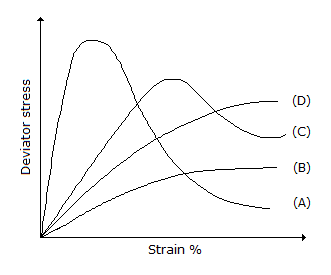# Civil Engineering - UPSC Civil Service Exam Questions

31.

Consider the following statements :
Bearing stiffness, in plate girders, are :
1. provided at supports.
3. provided alternately on the web.
Which of the statements given above is/are correct ?

 A. 1 only B. 2 only C. 1 and 2 D. 1, 2 and 3

Explanation:

No answer description available for this question. Let us discuss.

32.

Which one of the following statements is correct ?
As the depth of immersion of a vertical plane surface increases, the location of centre of pressure.

 A. comes closer to the centre of the area B. moves apart from the centre of gravity of the area C. ultimately coincides with the centre of gravity of the-area D. remains unaffected

Explanation:

No answer description available for this question. Let us discuss.

33.

A highly sensitive undisturbed clay sample was brought to the laboratory and UU test was performed. The curve of deviator stress versus strain % plot will be (among the four curves shown in the given figure) the one labelledA. A B. B C. C D. D

Explanation:

No answer description available for this question. Let us discuss.

34.

The sequent depth ratio in a hydraulic jump formed in a horizontal rectangular channel is 16.48. The flow is supercritical. What is the value of the Froude number of flow ?

 A. 4 B. 8 C. 12 D. 120

Explanation:

No answer description available for this question. Let us discuss.

35.

Consider the following operations in a spire test :
1. Depress telescope and sight a point on the ground nearer to the instrument
2. Clamp horizontal plates
3. Sight a well defined high point on a high building
4. Change face and repeat the procedure.
The correct sequence of these operations is

 A. 1, 2, 3, 4 B. 3, 1, 2, 4 C. 3, 2, 1, 4 D. 2, 1, 3, 4